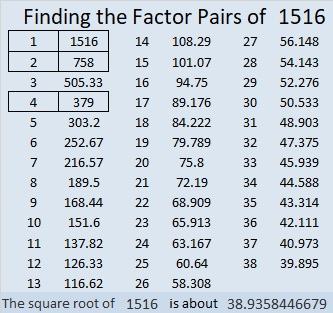# 1516 and Level 4

Contents

### Today’s Puzzle:

Using logic, write each number from 1 to 12 in both the first column and the top row so that those numbers are the factors of the given clues.### Factors of 1516:

• 1516 is a composite number.
• Prime factorization: 1516 = 2 × 2 × 379, which can be written 1516 = 2² × 379.
• 1516 has at least one exponent greater than 1 in its prime factorization so √1516 can be simplified. Taking the factor pair from the factor pair table below with the largest square number factor, we get √1516 = (√4)(√379) = 2√379.
• The exponents in the prime factorization are 2 and 1. Adding one to each exponent and multiplying we get (2 + 1)(1 + 1) = 3 × 2 = 6. Therefore 1516 has exactly 6 factors.
• The factors of 1516 are outlined with their factor pair partners in the graphic below.### One More Fact about the Number 1516:

Only one of its factor pairs add up to an even number, so 1516 is the difference of two squares in only one way:
380² – 378² = 1516.

This site uses Akismet to reduce spam. Learn how your comment data is processed.